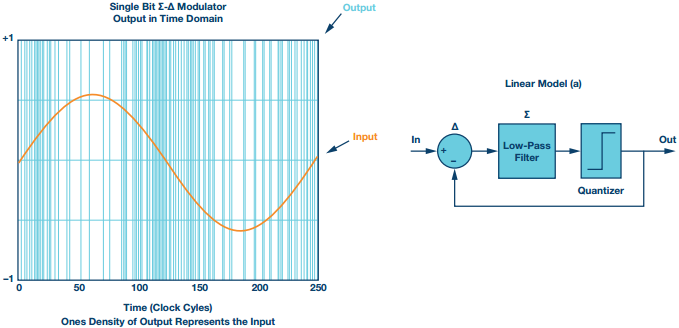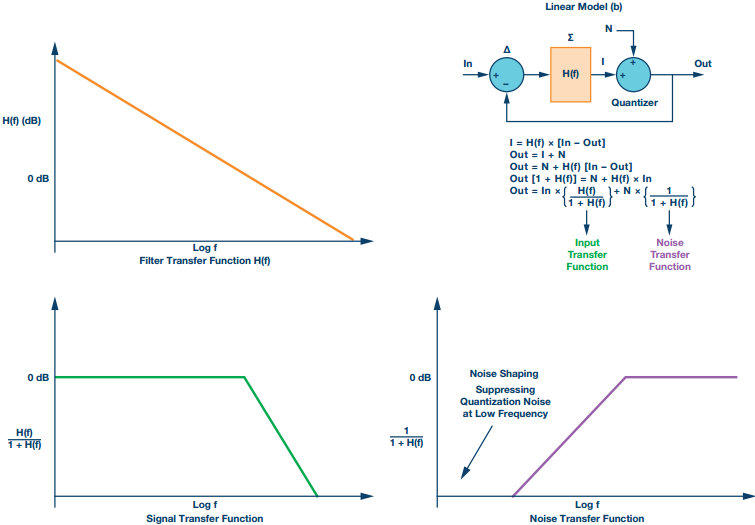# Fundamental Principles Behind the Sigma-Delta ADC Topology: Part 1

The Σ- ADC is a staple in the tool kit of today’s signal acquisition and processing system designers. The aim of this article is to give the reader the base knowledge on the fundamental principles behind the Σ- ADC topology. Examples of the trade-offs between noise, bandwidth, settling time, and all other key parameters associated with ADC subsystem design are explored to provide context for designers of precision data acquisition circuits.

Typically there are two blocks: the Σ- modulator and the digital signal processing block, usually a digital filter. This high level block diagram and the key concepts of the Σ- ADC are shown in Figure 1.

As the Σ- modulator is an oversampled architecture, let’s start with the sampling theory and the scenario of Nyquist and oversampled ADC operation.

Figure 2 illustrates the comparison between the Nyquist operation of an ADC with the oversampled case and finally with the Σ- modulated (also oversampled) case.

Figure 2a represents the quantization noise of an ADC when running in a straight Nyquist operation. In this case, the quantization noise is determined by the LSB size of the ADC. FS is the sampling rate of the ADC and FS/2 is the Nyquist frequency. Figure 2b shows the same converter, except now it is used in an oversampled context so a faster sampling rate is employed. The sampling rate is increased by a factor of K with the quantization noise now spread over a wider bandwidth up to K × FS/2. The low-pass digital filter (typically with decimation) removes quantization noise outside of the blue region.

The Σ- modulator has the added feature of noise shaping, as shown in Figure 2c. The quantization noise of the analog-to-digital conversion is shaped by the modulation scheme, shifting it (typically) from a low bandwidth up to a higher frequency, allowing a low-pass digital filter to eliminate it from the conversion result. The Σ- ADC can be designed with the noise floor determined by thermal noise and not limited by quantization noise.

### Sample, Modulate, Filter

The Σ- ADC is clocked using either an internal or external sampling clock. Often the ADC’s master clock (MCLK) is divided down prior to use by the modulator; be mindful of this when reading the ADC data sheet and understand the modulator frequency. The sampling frequency passed to the modulator sets the sampling frequency FMOD. The modulator outputs data to the digital filter at this rate, in turn the digital filter (typically low- pass, with some decimation) provides data at the output data rate (ODR). Figure 3 illustrates this flow.

### In-Depth View of a First Order Σ- Modulator

The Σ- modulator is a negative feedback system, analogous to a closed- loop amplifier. The loop contains a low resolution ADC and DAC, as well as a loop filter. The output and feedback are coarsely quantized often only a single bit output as a high or low. The basic structure is implemented as an analog system for ADCs, where the quantizer is the block in which the sampling is accomplished. Provided conditions exist for stability of the loop, the output is a coarse representation of the input. The digital filter takes the coarse output and reconstructs an accurate digital version of the analog input.

A ones density output in response to a sine wave input is illustrated in Figure 4. The rate of change of the modulator output from a low level to high level depends on the rate of change of the input. At full-scale input of the sine wave, the modulator output switching rate reduces and the output +1 state dominates. Similarly when the sine wave is at its negative full scale, the transitions between +1 and –1 are reduced and the –1 output dominates. At the maximum rate of change of the sine wave input, the highest density of switching between +1 and –1 in the modulator output occurs. The rate of change of the output is following that of the input. It is the rate of transition of the Σ- modulator output that describes the analog input.Figure 4. Σ-Δ ones density in response to sine wave input. Linear model (a) of the Mod 1 Σ-Δ loop.

Using a linear model to describe this single bit modulator (Mod 1) the system is shown as a control system with negative feedback. The quantization noise is the difference between the input and the output of the quantizer. A low-pass filter follows the input delta node. In Figure 5b, the quantization noise is described by the term N.Figure 5. Linear model (b) of the Mod 1 Σ- loop including equations, filter, signal, and noise transfer function plots.

H(f) is the function of the loop filter and it defines both the noise and signal transfer functions. H(f) is a low-pass filter function with very high gain at low frequencies (within the bandwidth of interest) and attenuation of higher frequency signals. The loop filter can be implemented as a simple integrator or a cascade of integrators. In practice a DAC is placed in the feedback path to take the digital output signal and feed it back to the analog input delta node.

Solving the equations shown in Figure 5 gives the signal and noise transfer functions. The signal transfer function operates as a low-pass filter, with a gain of 1 in the bandwidth of interest. The noise transfer function is a high- pass filter function, providing the noise shaping. There is strong suppression of the quantization noise at low frequencies around dc. The quantization noise signal seen at high frequencies outside the bandwidth of interest is increased. For the single-order modulator (Mod 1), the noise increases at a rate of approximately 20 dB/decade.

A common method to increase the resolution of the system is to increase the loop filter order by cascading two loop filters. The H(f) of the overall loop filter now has a greater roll off, and the noise transfer function has a transition of 40 dB/decade for a Mod 2 style. The quantization noise is shaped more aggressively, with much lower frequency noise. Figure 6 compares Mod 1 and Mod 2 Σ- ADCs. The variations and styles of Σ- modulators are wide ranging. Architectures that circumvent stability concerns of higher order, single bit loops are called multistage noise shaping modulators (MASH) architectures. The multistage (MASH style) architectures enable design of stable, high order Σ- modulators through a combination of inherently stable lower order loops.Figure 6. Mod 1and Mod 2 block diagram configurations with comparative plots of the filter and noise transfer functions.